## Monday, November 28, 2016

### SCIENCE! With Scott: Competition

(Click on the comic if you can't see the full image.)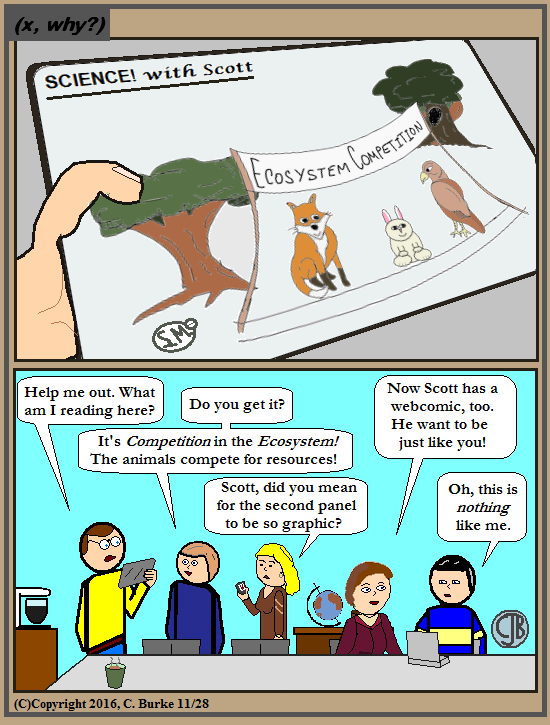There's a learning curve for new webcomic writers, even if they're teachers, too.

I like doing the occasional Science comic, but I don't know if this feature in particular will be ongoing. What do you think? (What's your vote?) By the way, I decided on the teacher's name a while ago, even if I haven't used it. (He has a last name, too.)

Ken's comic should be making a comeback at some point. Problem is that I have to rework all of them, and put a little bit more of my style (or one of my styles, at any rate) into them.

Come back often for more funny math and geeky comics.## Sunday, November 27, 2016

### Volume Displacement

(Click on the comic if you can't see the full image.)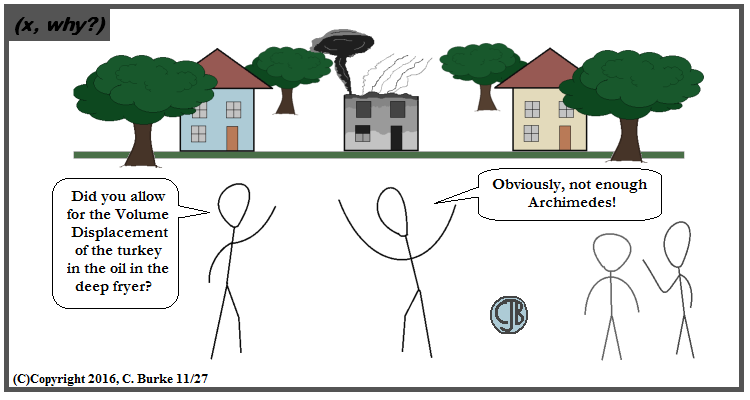One method: Put the turkey in first. Fill the fryer with oil. Remove turkey. Heat the oil.

This one should've been up the day after Thanksgiving, except a) wasn't ready yet because we were enjoying the holiday, and b) I was a little worried that the newspaper would be filled with tragedy from this happening, despite it being a sitcom staple.

I hope everyone's Thanksgiving was a happy and safe one. I'm having great memories of Leftover Sundays past.

Come back often for more funny math and geeky comics.## Wednesday, November 23, 2016

### Thanksgiving Probability Problem

(Click on the comic if you can't see the full image.)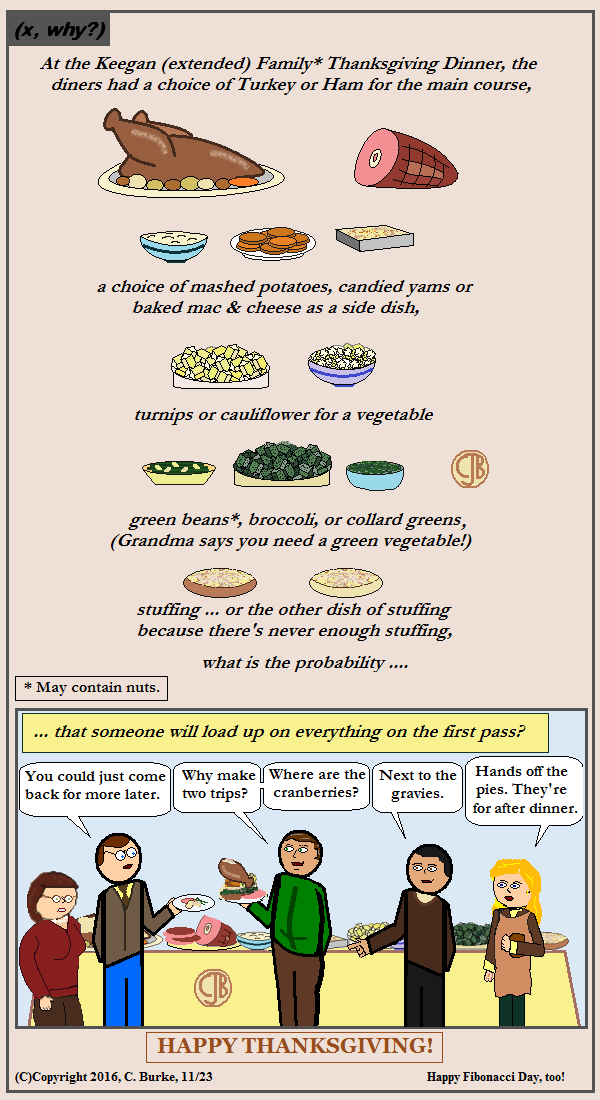It approaches 1 as more people approach the buffet.

Unlike a previous Thanksgiving with the Family comic, I didn't try to squeeze everyone into this "extended" family gathering. However, Thanksgivings are big affairs. Now that the torch has passed from mother to daughter (who is married, so nee Keegan), there are more guests with nieces and nephews and assorted relatives who are alone for the holiday because their immediate families no longer live around here.

Yeah, there are a lot of complaints, and sometimes it gets a little heated -- the oven has been on all day, after all -- it's still family. They're the only ones you have. And when they're gone, they're gone.

Come back often for more funny math and geeky comics.## Saturday, November 19, 2016

### June 2016 Common Core Algebra II Regents, Part 2

I don't usually post the answers to the Algebra II exam, but I've received enough requests that I've decided to post some of the open-ended questions.

### June 2016, Algebra II

25. Solve for x: (1 / x) - (1 / 3) = -(1 / 3x)

The answer is best given in picture form, shown below. Basically, you need to find a common denominator to combine the fractions. You can then solve by cross-multiplying.
Alternatively, you could just multiply all the terms by 3x because x, 3, and 3x are all factors of 3x. The multiplication would remove all the fractions from the problem.

x = 4.

26. Describe how a controlled experiment can be created to examine the effect of ingredient X in a toothpaste.

This was really is open-ended. You can write a lot of different things, but there are a few points which will be mandatory. Basically, you needed to have a number of people, (pick a number, say 10 or 20), and assign them randomly to two groups. One of these groups will try the new toothpaste, and the other is the control group which will not use the new toothpaste.

27. Determine if x - 5 is a factor of 2x3 - 4x2 - 7x - 10. Explain your answer.

One way to figure out if it is a factor is to divide the polynomial by (x - 5). If there is no remainder, then it is a factor.
Another is to say, if x - 5 is a factor of the polynomial, then 2x3 - 4x2 - 7x - 10 = 0 when x = 5. Substitute 5 for x and see if you get 0. (You won't.)

The image below shows the result of the division. It does not divide evenly. There is a remainder. So it is NOT a factor.

28. On the axes below, graph one cycle of a cosine function with amplitude 3, period pi/2, midline y = -1, and passing through the point (0,2).

You needed several things for this two points. You would lose 1 point for one mistake, and 2 points for 2 or more mistakes.
You needed to have a scale to the graph. It needed to pass through (0, 2) and have the proper midline (y = -1)

29. A suburban high school has a population of 1376 students. The number of students who participate in sports is 649. The number of students who participate in music is 433. If the probability that a student participates in either sports or music is 974/1376, what is the probability that a student participates in both sports and music

The total number of students who play either sports or music is equal to the number who play sports PLUS the number who play music MINUS the ones who play BOTH. If you just add the first two numbers, then you will double count the students who participate in both activities.

You are given the total that play one or the other. You need to calculate the number who play both. Add the two numbers (649 + 433) and subtract 974 from that sum of 1082, which gives you 108. However, since this is a probability question, you need to write it as a fraction of the student population, or 108/1376.

The image shows what to do:

30. The directrix of the parabola 12(y + 3) = (x - 4)2 has the equation y = -6. Find the coordinates of the focus of the parabola.

The directrix of a parabola is a horizontal line running below the vertex. (Or above, if it opens down.) The focus is a point such that the distance from it to the parabola is equal to the vertical distance from the parabola to the directrix.

To find the coordinates of the focus, you need to find the coordinates of the vertex, which would be exactly in the middle on a straight line. The focus will have the same x-coordinate as the vertex. The focus will be as far above the vertex as the directrix is below.

Rewrite the equation 12(y + 3) = (x - 4)2
Divide both sides by 12: y + 3 = (1/12)(x - 4)2
Subtract 3 from both sides: y = (1/12)(x - 4)2 - 3
The vertex (h, k) is at (4, -3)
The directrix is y = -6. (-6) - (-3) = 3.
Add 3 to the vertex y-coordinate: (-3) + 3 = 0, which must be the y-coordinate of the focus.
The focus is at (4, 0).

31. Algebraically prove that (x3 + 9)/(x3 + 8) = 1 + (1)/(x3 + 8), where x =/= -2.

Change 1 into (x3 + 8)/(x3 + 8). Do the addition. The left and right sides are equal.

32. A house purchased 5 years ago for \$100,000 was just sold for \$135,000. Assuming exponential growth, approximate the annual growth rate, to the nearest percent.

Set up the compound interest equation, A = P(1 + r/n)^(nt).
Substitute what you know, A = 135,000, P = 10000, n = 1 (annual), t = 5 years: 135000 = 100000(1 + r/1)^((1)(5))
Solve for r:

135000 = 100000(1 + r)^5
1.35 = (1 + r)^5
1.35^(1/5) = 1 + r
1.06185875879 = 1 + r
0.06185875879 = r

r = 6%

End of Part II

How did you do?
Comments, questions, corrections and concerns are all welcome.
Typos happen.

## Monday, November 14, 2016

### Distributive Property

(Click on the comic if you can't see the full image.)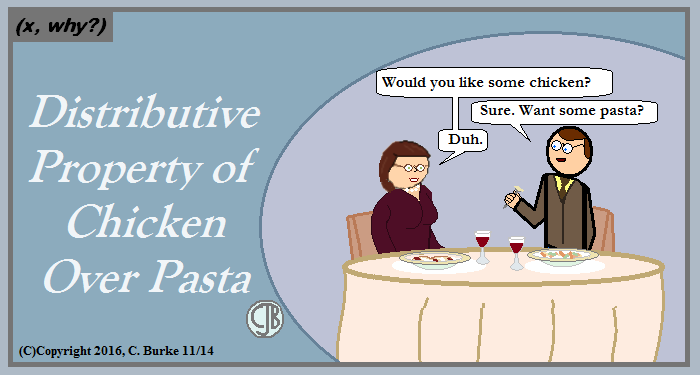And both plates become equal or balanced or delicious or something. Waiter, more bread, please!

Come back often for more funny math and geeky comics.## Friday, November 11, 2016

### Happy Veterans Day 2016

(Click on the comic if you can't see the full image.)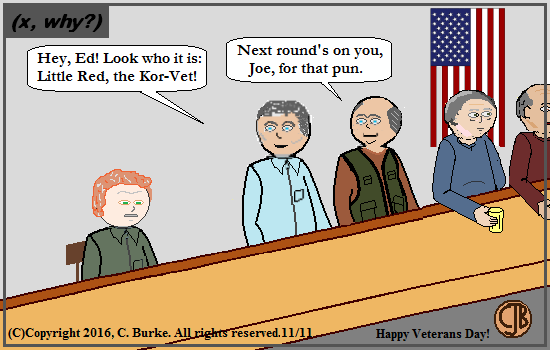He's a prince of a guy.

Happy Veterans Day

Come back often for more funny math and geeky comics.